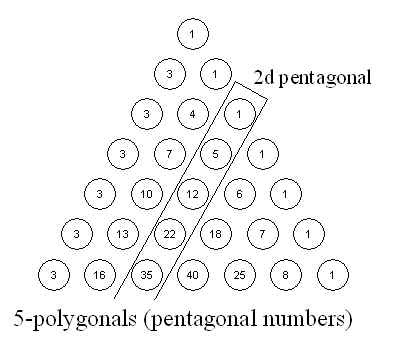## Thursday, February 19, 2009

### A Family of Sequences and Number Triangles

As mentioned in a previous post, the triangular numbers (and higher triangular numbers) can be generated using this recurrence relationship:These will form Pascal’s triangle (if we shift the variables n->n+d-1 and d->r, we get the familiar C(n,r) indexing for the Pascal Triangle). The d=2 case gives the usual “flat” 2d triangular numbers, and other d values provide triangular numbers of different dimensions.

It turns out that recurrence relation can be generalized to generate a family of sequences and triangles. Consider this more general relation:

Doing some initial exploring reveals four interesting cases:

The triangular numbers
With all these additional parameters set to 1, we get our original relation, the familiar triangular numbers, and Pascal’s triangle.The k-polygonal numbers
If we set the “zero dimension” to k-2, we end up with the k-polygonal numbers (as described here). The triangular numbers arise in the special case where k=3. Except in the k=3 case, the triangles that are generated are not symmetrical.

Below is the triangle generated by setting k=5.The symmetrically shifted k-polygonal numbers
As far as I know, there is not a standard name for these.  Each k value will generate a triangle that is symmetrical about its center and whose edge values are equal to k-2. For a given k value, if you enter sequences generated by particular values of d into the Online Encyclopedia of Integer Sequences you’ll find that some are well known. The codes in the diagrams correspond to the sequence ids from the Encyclopedia.

Here is the triangle generated by k=4:

And here is the triangle generated for k=5:

The Eulerian numbers (Euler’s number triangle)
This is a particularly nice way to generate the Eulerian numbers, which have a nice connection to the triangular numbers (see this post). There is a little inconsistency in the way the Eulerian numbers are indexed, however. For this formula to work, it should be altered slightly so that d>0. The resulting formula looks like this:

And the triangle looks like this:

It is surprising that so many interesting and well known sequences and triangles can be generated from such a simple formula, and that they can be interpreted as being part of a single family.